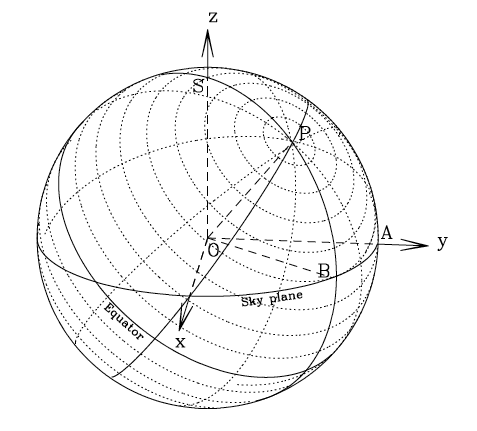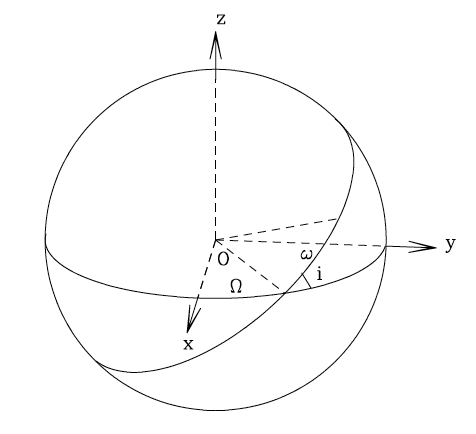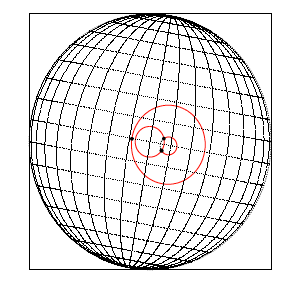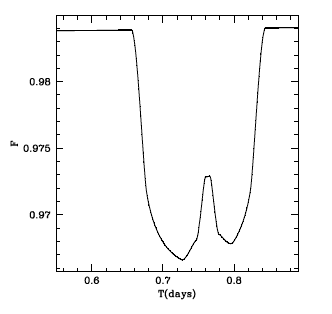## 1. Preface

KS integration (from Kelvin-Stokes integration) is a software that permits lightcurve modelling in presence of stellar activity, able to account for the photometric effect generated by transiting planets and stellar spots, resolving also the cases in which these objects appear overlapped with respect to the observer. It allows spot merging and the creation of spot regions.

## 2. Licence

This program is free software: you can redistribute it and/or modify it under the terms of the GNU General Public License as published by the Free Software Foundation, either version 3 of the License, or any later version.

This program is distributed in the hope that it will be useful, but WITHOUT ANY WARRANTY; without even the implied warranty of MERCHANTABILITY or FITNESS FOR A PARTICULAR PURPOSE. See the GNU General Public License for more details.

You should have received a copy of the GNU General Public License along with this program. If not, see http://www.gnu.org/licenses/

Contributed by M. Montalto, G. Boué, M. Oshagh, I. Boisse, G. Bruno, N. C. Santos

## 3. Installation

The software is written in Fortran 95 and it has been tested under a Linux machine using the GNU Fortran compiler gfortran. The following instructions hold under the assumption that you have this compiler already installed in your machine. To proceed first unpack the tarfile:

tar -xvf KSint.tar.gz

this will create a directory KSint. Enter this directory and to create the executable type:

make

the executable KSint will be therefore found under the same directory. This program is a standalone program that works together with the input file 'inputs.txt' also located in the same directory as explained in the next Section.

In case you prefer to supply your own inputs in a different form or just want to incorporate this software in your own programs the main program under the SRC directory (KSint.f95) can be inspected. The subroutine that performs all the calculations is named KSflux.

## 4. Input file

Table 1 lists input parameters provided to the program through the input file 'inputs.txt' in their order of appearance. Input parameters are subdivided in four groups: SIMPARAM contains parameters relative to the simulation, STARPARAM parameters relative to the star, SPOTS and PLANETS relative to spots and planets respectively as indicated by their name. For these two latter groups the parameters relative to different planets (spots) are provided as a comma separated list where it is only the number of planets and spots declared by 'nplanets' and 'nspots' that will be considered during program execution.

Table 1-Input parameters located in the file 'inputs.txt'.
• Name
• Type
• Units
• Bounds
• Meaning
• SIMPARAM

• timestep
• REAL
• [days]
• >0.
• Timestep of each iteration
• nstep
• INTEGER
• -
• ≥1
• Total number of iterations
• nplanets
• INTEGER
• -
• ≥0
• Number of planets
• nspots
• INTEGER
• -
• ≥0
• Number of spots
• STARPARAM

• prot
• REAL
• [days]
• >0.
• Stellar rotation period
• incl
• REAL
• [degrees]
• [0.;180.]
• Stellar axis inclination
• posang
• REAL
• [degrees]
• [0.;360.]
• Position angle of stellar axis
• rho
• REAL
• [gcm-3]
• >0.
• Stellar density
• c1
• REAL
• -
• [0.;1.]
• Linear limb darkening coefficient
• c2
• REAL
• -
• [0.;1.]
• SPOTS

• lat
• REAL,REAL,...
• [degrees]
• [-90.;90.]
• Spots latitudes
• long
• REAL,REAL,...
• [degrees]
• [0.;360.]
• Spots longitudes
• REAL,REAL,...
• [degrees]
• ]0.;180.]
• Spots angular dimensions
• ffact
• REAL,REAL,...
• -
• ≠0,≤1.
• Spots contrast ratios
• PLANETS

• rp
• REAL,REAL,...
• -
• >0.
• porb
• REAL,REAL,...
• [days]
• >0.
• Planets orbital periods
• pincl
• REAL,REAL,...
• [degrees]
• [0.;180.]
• Planets orbital inclinations
• ecc
• REAL,REAL,...
• -
• [0.;1.[
• Planets orbital eccentricities
• w
• REAL,REAL,...
• [degrees]
• [0.;360.]
• Planets arguments of pericenters
• omega
• REAL,REAL,...
• [degrees]
• [0.;360.]
• Planets longitudes of the ascending nodes
• M
• REAL,REAL,...
• [degrees]
• [0.;360.]
• Planets initial mean anomalies

## 5. Reference systems

The angles introduced in Table 1 for planets and spots are defined in Figure 1 and Figure 2. The plane xy corresponds to the plane of the sky, while the z-axis is oriented towards the observer.

Figure 1 - Star reference system.In Figure 1, the star reference system is defined. The angle between the North polar axis, from which the rotation is seen counter-clockwise, and the line of sight (the arc SP) indicates the inclination angle of the stellar axis (incl parameter). The angle between the positive y-axis and the projection of the stellar axis to the plane of the sky (the arc AB) is defined as the position angle of the stellar axis (posang parameter). The latitude of a spot (lat) is defined along a stellar parallel from the equator, positive towardsthe North pole. The longitude of a spot is measured along a stellar meridian where the zero longitude meridian is the one passing through the positive x-axis.

Figure 2 - Planets reference system.Figure 2 defines the planets reference system. In this case the inclination ofthe orbital plane (pincl), the argument of periastron (w) and the longitude of the ascending node (omega) are indicated in Figure 2 respectively by i, ω and Ω.

## 6. Overlapping spots

The present version of the code assumes the following rules once two or more spots are overlapped: two or more spots with the same contrast ratio will merge homogeneously (forming a region with the same contrast ratio of the components), while two of more spots with different contrast ratios will produce a region where the contrast ratio in the overlapping area will be the sum of the contrast ratio of the components. Note that it is not allowed to overlap two spots if the sum of their contrast ratios is larger than one.

## 7. Results

The results are stored by default in the file 'lc.dat'. Optionally the program can reproduce the configuration of the system at any given iteration. To enable this feature you should call the program with the '-v niter' option where niter is the iteration number you want to analyze. If the iteration number is not provided the program will reproduce by default the first iteration configuration. The result is stored in the file 'arcs.dat'.

The file 'lc.dat' contains three columns which correspond to the time of each iteration, the flux calculated by the program and an error flag which is equal to zero if no errors were detected.

The file 'arcs.dat' is separated in three different sections. The first line provides the starting, final lines in the file relative to the ARCS section, and in the last column provides the number of objects to which the arcs belong. The second line provides the starting, final lines in the file relative to the stellar GRID section, and the third line gives the starting line of the ROOTS section.

All the coordinates reported below are expressed with respect to the XY observer plane.

The ARCS section provides the coordinates x, y of each point on a given arc, followed by a flag which is one if that point lies on an integrable arc, and by a number which is unique for each object (one corresponds by default to the stellar border). The GRID section gives the x, y coordinates of each point on a stellar parallel or meridian. The third column is just an incremental number running along the maximum circles, while the last column is a flag which is equal to one in the case the point belongs to a parallel, two if it belongs to a meridian and zero if it lies on the invisible hemisphere of the star. The ROOTS section gives the x, y coordinates of the arcs interception points found by the root finding algorithm. A fully integrable object has always a root attributed to the arc defining its profile, since the arc is integrated between zero and 2π.

Under the directory SM and GNUPLOT simple macros are provided and can be used to visualize the results in 'lc.dat' and 'arcs.dat'.

Additionally, once the '-v niter' option is used an arc table is printed on the screen, showing, for each object, the list of arcs splitting the profile of each object. The starting and final angles of the arc are reported in the first and second columns respectively. For the case of planets and the case of the stellar border, the angle is counted counter-clockwise from the positive x-axis with respect to a reference system centered on each object and with the axis oriented like the observer XY reference system. For the case of spots the angle α is reported as defined in Fig. 1 of the paper. The third column gives the contrast ratio associated to the arc (this is the factor that multiplies the integral on that arc, resulting from the analysis of the structure), while the fourth column is a flag indicating if the arc is considered integrable (in this case the flag is equal to one).

## 8. Example

The following example may be used as a guide to get started using the program. We simulate the case of a planet crossing a naked eye spot. The input parameters are provided in Table2. The resulting configuration (as seen by the observer) at iteration 833 of the simulation is shown in Figure 3. Here the positive x-axis is oriented towards the right while the positive y-axis towards the top. The resulting lightcurve is shown in Figure 4.

Table 2-Input parameters relative to the example
discussed in the text.
• Name
• Value
• SIMPARAM

• timestep
• 0.0009
• nstep
• 1000
• nplanets
• 1
• nspots
• 2
• STARPARAM

• prot
• 30.
• incl
• 90.
• posang
• 10.
• rho
• 0.4
• c1
• 0.4
• c2
• 0.3
• SPOTS

• lat
• 0.2,-0.3
• long
• 270.2,270.
• 18.,4.
• ffact
• 0.1,0.8
• PLANETS

• rp
• 0.12
• porb
• 3.
• pincl
• 90.
• ecc
• 0.
• w
• 0.
• omega
• 180.
• M
• 0.
Figure 3 - Planet overlapped with a naked eye spot at iteration 833 of the example.Figure 4 - This Figure shows a portion of the resulting lightcurve where the planet overlaps the spots.## 9. Credits

The KS technique applied to the case of planets and spots is discussed in the two following papers:

Pál, A. 2012, MNRAS, 420, 1630

Montalto, M., Boué, G., Oshagh, M., Boisse, I., Bruno, G., Santos, N. C. 2014, MNRAS, 444, 1721

The solution presented in this software incorporates the one on planets presented in Pál, therefore any work where this software is used should mention these two papers.